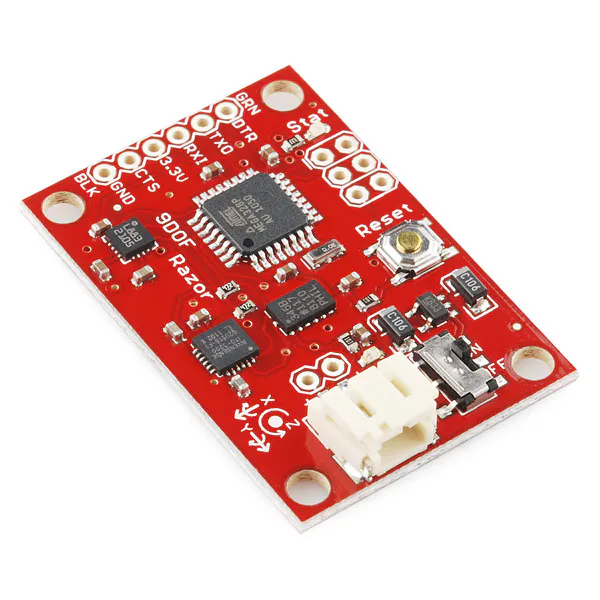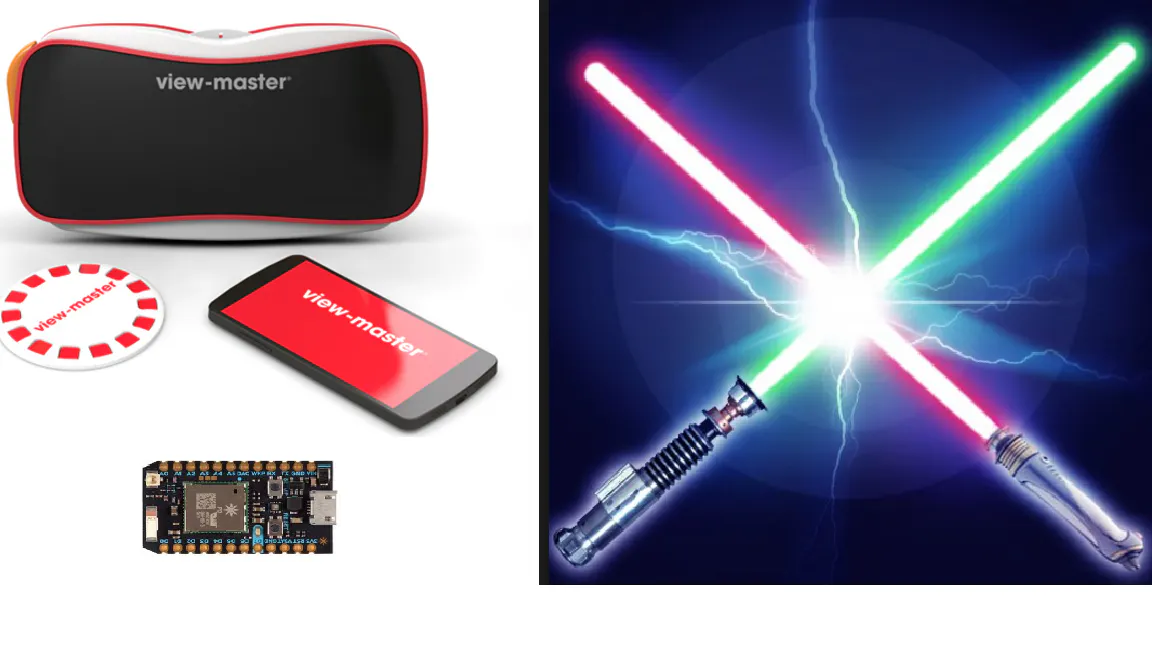# Photon Lightsaber Controller for VR/AR

Ever wanted to build your own Lightsaber? You can create your own LightSaber controller in VR using Photon to learn the Jedi ways.

IntermediateFull instructions provided4,987

## Things used in this project

### Hardware componentsParticle Photon
×1SparkFun 9 Degrees of Freedom - Razor IMU
×1
 lipstick size power bank
×1

### Software apps and online servicesUnity

## Schematics

### ViewMasterSaber.png## Code

### imu-udp.ino

Arduino
I used Particle IDE, I added the Kalman/Kalman.h library
```// This #include statement was automatically added by the Particle IDE.
#include "Kalman/Kalman.h"

#include "SparkFunLSM9DS1/SparkFunLSM9DS1.h"
#include "math.h"

//////////////////////////
// LSM9DS1 Library Init //
//////////////////////////
// Use the LSM9DS1 class to create an object. [imu] can be
// named anything, we'll refer to that throught the sketch.
LSM9DS1 imu;

///////////////////////
// Example I2C Setup //
///////////////////////
// SDO_XM and SDO_G are both pulled high, so our addresses are:
#define LSM9DS1_M   0x1E // Would be 0x1C if SDO_M is LOW
#define LSM9DS1_AG  0x6B // Would be 0x6A if SDO_AG is LOW

////////////////////////////
// Sketch Output Settings //
////////////////////////////
#define PRINT_CALCULATED
//#define PRINT_RAW
#define PRINT_SPEED 10 // 250 ms between prints

// Earth's magnetic field varies by location. Add or subtract
// a declination to get a more accurate heading. Calculate
// your's here:
// http://www.ngdc.noaa.gov/geomag-web/#declination
//#define DECLINATION -8.58 // Declination (degrees) in Boulder, CO.

#define DECLINATION -3.42 // Declination (degrees) in Fort Worth.

int i = 0;
// An UDP instance to let us send and receive packets over UDP
UDP Udp;

uint8_t server[] = { 192, 168, 43, 1}; // ip address of my phone

unsigned int localPort = 33333;

uint32_t timer;

void setup()
{

Serial.begin(115200);

sprintf(myIpAddress, "%d.%d.%d.%d", myIp, myIp, myIp, myIp);

// start the UDP
Udp.begin(localPort);

// Before initializing the IMU, there are a few settings
// we may need to adjust. Use the settings struct to set
// the device's communication mode and addresses:
imu.settings.device.commInterface = IMU_MODE_I2C;
// The above lines will only take effect AFTER calling
// imu.begin(), which verifies communication with the IMU
// and turns it on.
if (!imu.begin())
{
Serial.println("Failed to communicate with LSM9DS1.");
Serial.println("Double-check wiring.");
Serial.println("Default settings in this sketch will " \
"work for an out of the box LSM9DS1 " \
"Breakout, but may need to be modified " \
"if the board jumpers are.");
while (1)
;
}

timer = micros();

}

void loop()
{

printAttitude(imu.ax, imu.ay, imu.az, -imu.my, -imu.mx, imu.mz, imu.gx, imu.gy, imu.gz);

//printGyro();

delay(PRINT_SPEED);
}

void printGyro()
{
// To read from the gyroscope, you must first call the
// readGyro() function. When this exits, it'll update the
// gx, gy, and gz variables with the most current data.

// Now we can use the gx, gy, and gz variables as we please.
// Either print them as raw ADC values, or calculated in DPS.
Serial.print("G: ");
#ifdef PRINT_CALCULATED
// If you want to print calculated values, you can use the
// calcGyro helper function to convert a raw ADC value to
// DPS. Give the function the value that you want to convert.
Serial.print(imu.calcGyro(imu.gx), 2);
Serial.print(", ");
Serial.print(imu.calcGyro(imu.gy), 2);
Serial.print(", ");
Serial.print(imu.calcGyro(imu.gz), 2);
Serial.println(" deg/s");
#elif defined PRINT_RAW
Serial.print(imu.gx);
Serial.print(", ");
Serial.print(imu.gy);
Serial.print(", ");
Serial.println(imu.gz);
#endif

Udp.beginPacket(IPfromBytes, localPort);

Udp.endPacket();
}

void printAccel()
{
// To read from the accelerometer, you must first call the
// readAccel() function. When this exits, it'll update the
// ax, ay, and az variables with the most current data.

// Now we can use the ax, ay, and az variables as we please.
// Either print them as raw ADC values, or calculated in g's.
Serial.print("A: ");
#ifdef PRINT_CALCULATED
// If you want to print calculated values, you can use the
// calcAccel helper function to convert a raw ADC value to
// g's. Give the function the value that you want to convert.
Serial.print(imu.calcAccel(imu.ax), 2);
Serial.print(", ");
Serial.print(imu.calcAccel(imu.ay), 2);
Serial.print(", ");
Serial.print(imu.calcAccel(imu.az), 2);
Serial.println(" g");
#elif defined PRINT_RAW
Serial.print(imu.ax);
Serial.print(", ");
Serial.print(imu.ay);
Serial.print(", ");
Serial.println(imu.az);
#endif

}

Kalman kalmanX; // Create the Kalman instances
Kalman kalmanY;
double gyroXangle, gyroYangle; // Angle calculate using the gyro only
double compAngleX, compAngleY; // Calculated angle using a complementary filter
double kalAngleX, kalAngleY; // Calculated angle using a Kalman filter

// Calculate pitch, roll, and heading.
// Pitch/roll calculations take from this app note:
// http://cache.freescale.com/files/sensors/doc/app_note/AN3461.pdf?fpsp=1
// Heading calculations taken from this app note:
void printAttitude(
float ax, float ay, float az, float mx, float my, float mz, float gx, float gy, float gz)
{
float roll = atan2(ay, az);
float pitch = atan2(-ax, sqrt(ay * ay + az * az));

if (my == 0)
heading = (mx < 0) ? 180.0 : 0;
else

heading -= DECLINATION * M_PI / 180;

double dt = (double)(micros() - timer) / 1000000; // Calculate delta time
timer = micros();

// Convert everything from radians to degrees:
pitch *= 180.0 / M_PI;
roll  *= 180.0 / M_PI;

double gyroXrate = gx / 131.0; // Convert to deg/s
double gyroYrate = gy / 131.0; // Convert to deg/s

if ((pitch < -90 && kalAngleY > 90) || (pitch > 90 && kalAngleY < -90)) {
kalmanY.setAngle(pitch);
compAngleY = pitch;
kalAngleY = pitch;
gyroYangle = pitch;
} else
kalAngleY = kalmanY.getAngle(pitch, gyroYrate, dt); // Calculate the angle using a Kalman filter

if (abs(kalAngleY) > 90)
gyroXrate = -gyroXrate; // Invert rate, so it fits the restriced accelerometer reading
kalAngleX = kalmanX.getAngle(roll, gyroXrate, dt); // Calculate the angle using a Kalman filter

double accelX = imu.calcAccel(ax);
double accelY = imu.calcAccel(ay);
double accelZ = imu.calcAccel(az);

Udp.beginPacket(IPfromBytes, localPort);

Udp.endPacket();

}
```

C#
Here's what I used to receive data in Unity3d. I attached this to a GameObject. It looks for another game object called sword (which is the lightsaber) and modifies it's rotation
```// ------------------------------------------------------------------------------
/*
-----------------------
-----------------------
// [url]http://msdn.microsoft.com/de-de/library/bb979228.aspx#ID0E3BAC[/url]

// 127.0.0.1 : 8051

// send
// nc -u 127.0.0.1 8051

*/
using UnityEngine;
using System.Collections;

using System;
using System.Text;
using System.Net;
using System.Net.Sockets;

public class UDPReceiveSaber : MonoBehaviour {

// udpclient object
UdpClient client;

// public
// public string IP = "127.0.0.1"; default local
public int port; // define > init
public float smooth = 1F;
public float tiltAngleX = 0.0F;
public float tiltAngleY = 0.0F;
public float tiltAngleZ = 0.0F;

public float accelX = 0.0F;
public float accelY = 0.0F;
public float accelZ = 0.0F;

public float velocityX = 0;
// infos
public string allReceivedUDPPackets=""; // clean up this from time to time!

string[] rot;

public GameObject lightSaber;
ParticleRenderer particleRenderer;

public GameObject sword;

// start from shell
private static void Main()
{

string text="";
do
{
}
while(!text.Equals("exit"));
}
// start from unity3d
public void Start()
{
init();

sword = GameObject.FindGameObjectWithTag ("Sword");
lightSaber = GameObject.FindGameObjectWithTag ("Light");
particleRenderer = lightSaber.GetComponentInChildren<ParticleRenderer> ();

}

// OnGUI
void OnGUI()
{
Rect rectObj=new Rect(40,10,200,400);
GUIStyle style = new GUIStyle();
style.alignment = TextAnchor.UpperLeft;
+ "shell> nc -u 127.0.0.1 : "+port+" \n"
,style);
}

// init
private void init()
{
// Endpunkt definieren, von dem die Nachrichten gesendet werden.
print("UDPSend.init()");

// define port
port = 33333;

// status
print("Sending to 127.0.0.1 : "+port);
print("Test-Sending to this Port: nc -u 127.0.0.1  "+port+"");

}

void Update () {

Quaternion target = Quaternion.Euler(- tiltAngleY, 0 ,-tiltAngleX);
sword.transform.localRotation = Quaternion.Slerp(sword.transform.localRotation, target,Time.deltaTime * 0.1);

}
{

client = new UdpClient(port);
while (true)
{

try
{
// Bytes empfangen.
IPEndPoint anyIP = new IPEndPoint(IPAddress.Any, 0);

// Bytes mit der UTF8-Kodierung in das Textformat kodieren.
string text = Encoding.UTF8.GetString(data);

// Den abgerufenen Text anzeigen.
print(">> " + text);

// latest UDPpacket

tiltAngleX = Convert.ToSingle (rot ); //roll
tiltAngleY = Convert.ToSingle (rot ); //pitch
tiltAngleZ = Convert.ToSingle (rot );
accelX = Convert.ToSingle (rot ) ; //roll
accelY = Convert.ToSingle (rot ) ; //pitch
accelZ = Convert.ToSingle (rot ) ;

}
catch (Exception err)
{
print(err.ToString());
}
}
}

// getLatestUDPPacket
// cleans up the rest
public string getLatestUDPPacket()
{